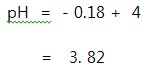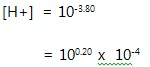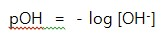# Advanced pH and pOH CalculationsIn my previous post you learned how to calculate the pH and the [H+] of solution even without the calculator using the formulaBut what about if the given Hydrogen Ion Concentration is not base of 1 like for example 0.00024 M and the pH is not a whole number like for example 7.5 pH.  You might say, use calculator.  Yes you are right calculator can be used.  But this post will calculate pH and [H+] without the use of calculator.

#### Converting [H+] to pH

In this type of calculation the same formula will be used only that additional steps will be added.Example,  a solution has a hydrogen concentration, [H+]  of  0.00015 M, what is the pH of the solution?

Solution:

The pH is expression isWe have to recall some rules in logarithm.  The logarithm of a product is equal to the sum of the logarithm of each value.  That is, log a x b = log a + log b .  Applying rule to the above equationNow its time to use logarithmic table as reference table for the logarithm of different numbers or you can have list of the logarithmic value of different numbers researched from the internet.
Continuing the calculation,This means that if a solution has hydrogen Ion Concentration of 0.00015 M has a pH of 3.82.

Another example:

Stomach acid has a Hydrogen Ion concentration, [H+] of  0.020 M.  What is the pH of the stomach acid?

Solution:### Converting pH to [H+]

In my previous blog [H+] is calculated usingLet us see for example the pH is 3.80,We have to remember the rule of exponent, to  multiply two exponential number, add the powers together. That is,.  Let us apply the rule on the above pH.
3.80 is the same as 0.20 + (-4).This time we need to use the antilogarithm table, 0.20 has antilog of 1.6, thereforeThis means that pH equal to 3.80 has a concentration of 0.000 16 M.

Another example:

Calculate the [H+] of seawater which has a pH of 7.85.

Solution:### pOH

What is pOH?  pOH expresses the basicity of the solution.  It is called the power of hydroxide.   The formula is the same with that of pH.The calculation is the same with pH and the [OH-].  Below is the summary between pH, pOH.TRY THIS:

Calculate the pH of the following  [H+]
1.  0.000 000 047 M
2.  0.000 089 M
3.  0.000 003 7 M

Calculate the [H+] given the following pH
1.  pH = 3.25
2.  pH = 8.68
3.  pH = 6.20

This post first appeared on Science Concepts And Questions (K To 12), please read the originial post: here